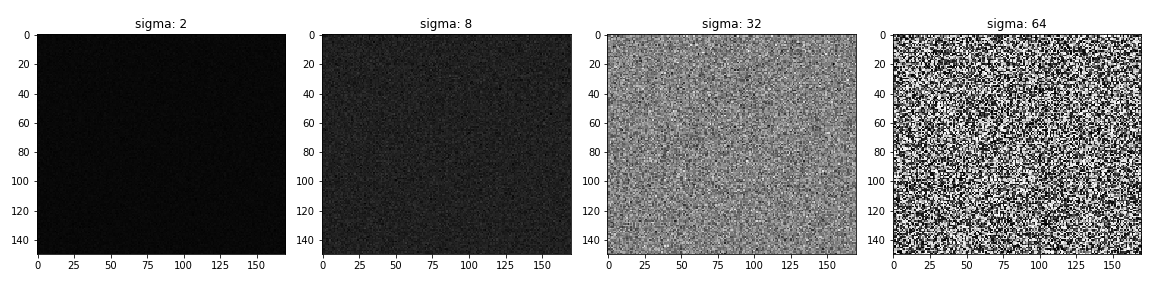# Images, filtering, convolution and edge detection

Contents

## Images as a functions

Images can be represented as a function: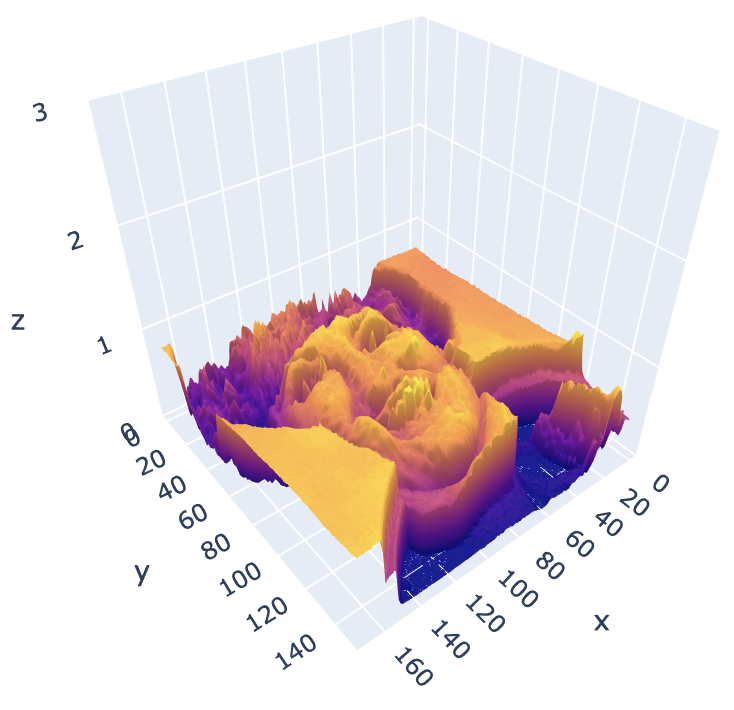We think of an image as a function, $f$ or $I$, from $\mathbb{R}^2$ to $\mathbb{R}$:

• $f(x, y)$ gives the intensity or value at position $(x,y)$.

A color image is just three functions “stacked” together. We can write this as “vector-valued function”:

\begin{equation*} f(x, y) = \begin{bmatrix} r(x, y) \\ g(x, y) \\ b(x, y) \end{bmatrix} \end{equation*}

In computers images are represented as a set of numbers, not continuous functions: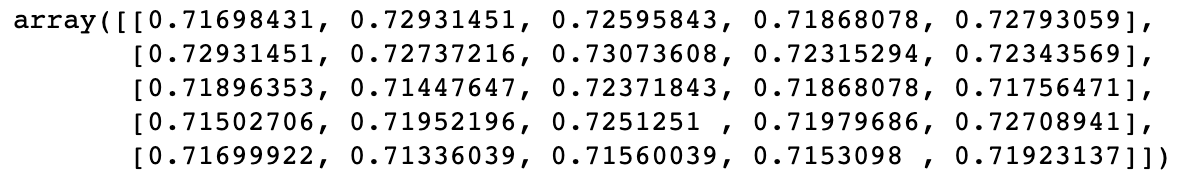In computer vision we typically operate on digital(discrete) images:

• Sample the 2D space on regular grid
• Quantize each sample (round to “nearest integer”)

## Noise

Noise is just another function that is combined with the original function to get a new one:

\begin{equation*} I’(x, y) = I(x, y) + \eta(x, y) \end{equation*}

Types:

• Impulse (salt): random occurrences of white pixels
• Pepper: random black pixels
• Salt and pepper: random occurrences of black and white pixels
• Gaussian noise: variations in intensity drawn from a Gaussian normal distribution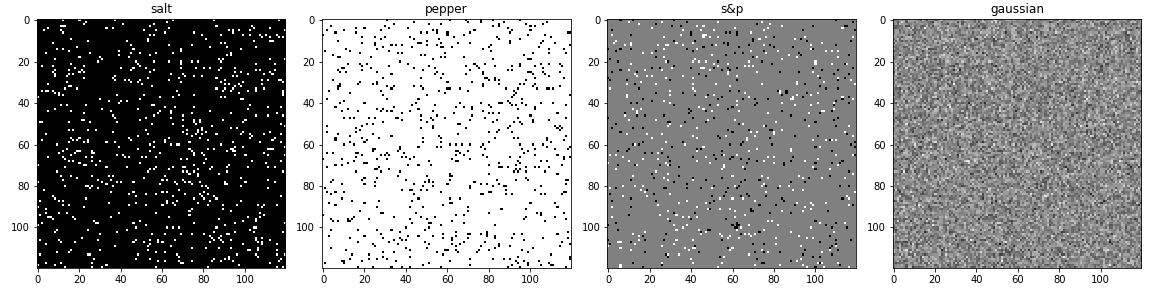To apply a noise it’s enough just to add it to the initial image:

noise = np.random.normal(mean, variance ** 0.5, image.shape)
output = image + noise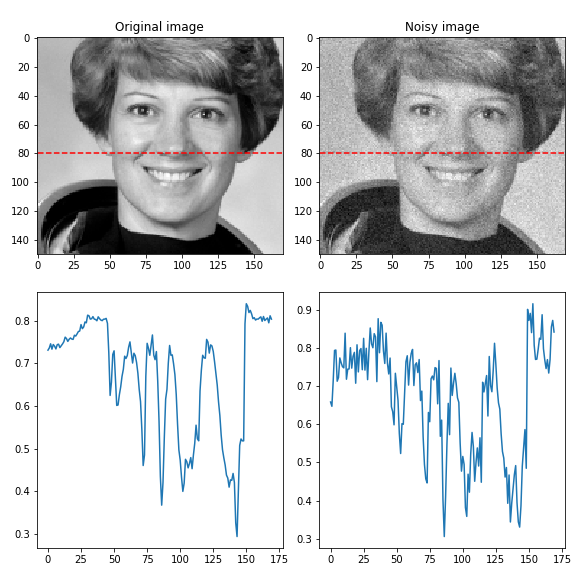Effect of $\sigma$ (standard deviation) on Gaussian noise. Just to remind: $variance = \sigma^2$.